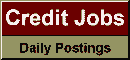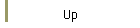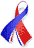DefaultRisk.com the web's biggest credit risk modeling resource.doi> search: A or B Export citation to:- HTML- Text (plain)- BibTeX- RIS- ReDIF

Are Default Correlations Time Dependent? A Bayesian approach

by Christina R. Niethammer of the University of Konstanz

June 30, 2008

Abstract: In this paper we discuss if asset correlations in a Gaussian one factor model are time dependent. Starting from S amd Ps default study, we examine a random factor loading type model (two correlations for two stages of the economy) and a model with time dependent variance of the common factor. As classical estimation is not very flexible, we apply Markov Chain Monte Carlo methods: An approach proposed by Wendin (2006) (Binomial approach) can be adopted to analyze a two stage correlation model. However an extended version of this Binomial algorithm becomes unstable in more complex models. We therefore develop an asset value approach to handle e.g. time dependence in the variance of the factor. Surprisingly, neither a two stage correlation nor a time dependence of the variance of the factor can be detected from loss rates.

Keywords: Bayesian, MCMC, asset value, default correlation, time dependence.

Previously titled: Are Asset Correlations Time Dependent? A Bayesian Approach

Books Referenced in this paper:  (what is this?)